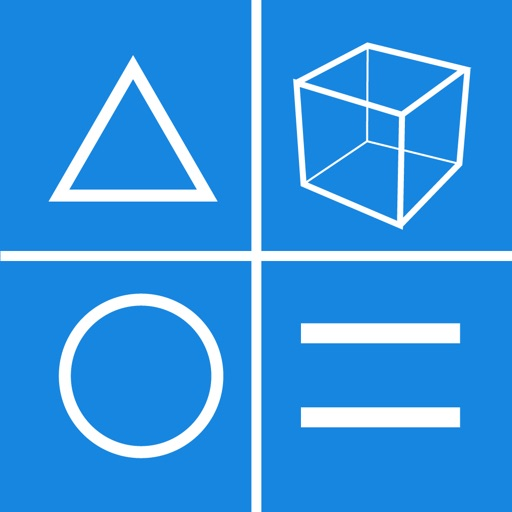## The Geometric Calculator is an app that makes calculations for a variety of geometric shapes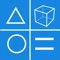# Geometric Calculator X

by Yoshiaki Onishi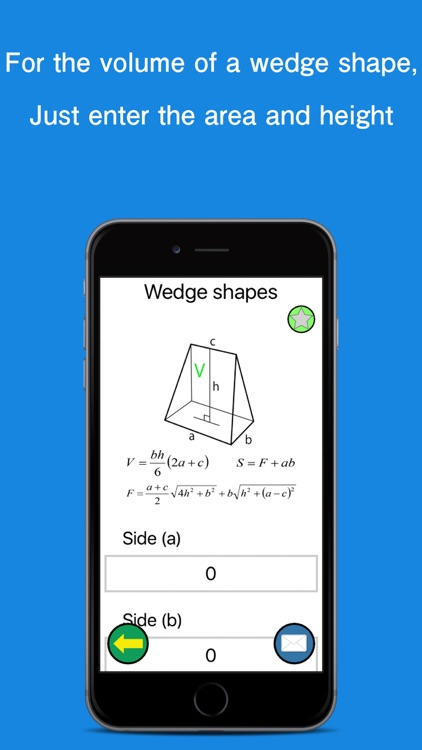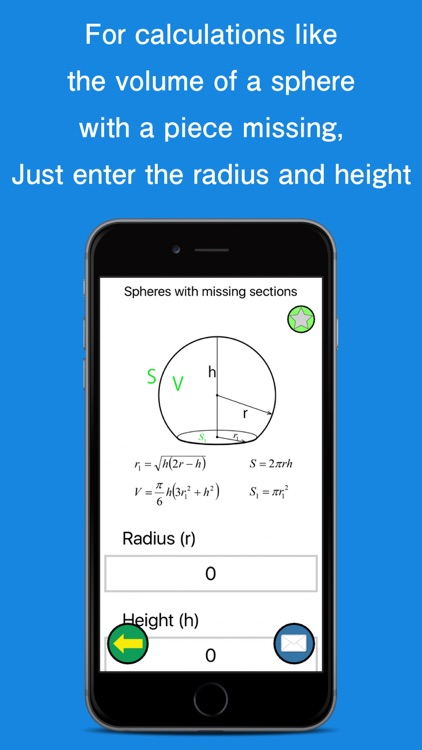The Geometric Calculator is an app that makes calculations for a variety of geometric shapes.### App Details

Version
1.5.5
Rating
(1)
Size
9Mb
Genre
Education Productivity
Last updated
January 14, 2019
Release date
January 15, 2016

### App Screenshots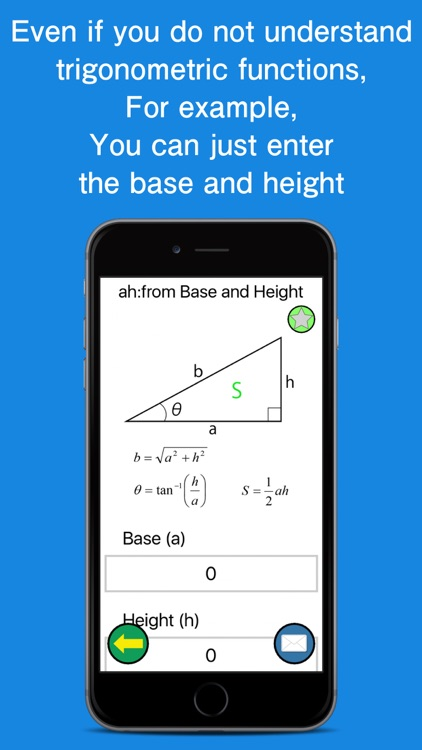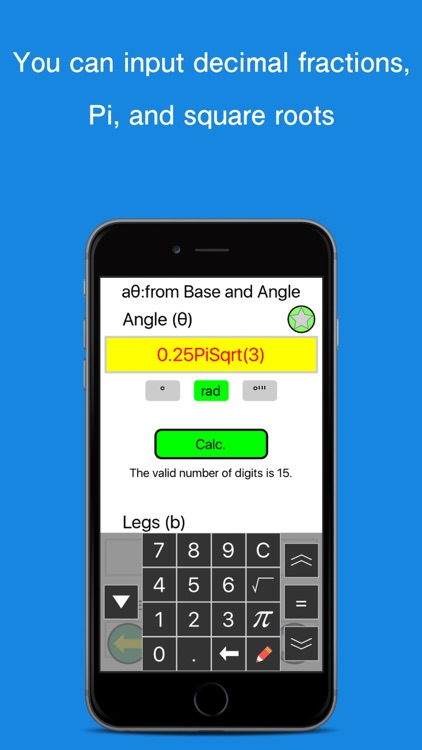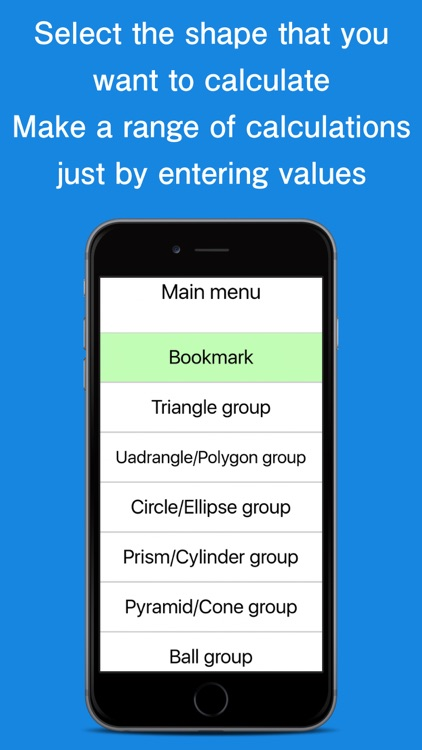### App Store Description

The Geometric Calculator is an app that makes calculations for a variety of geometric shapes.
It does not provide the functionality of a standard calculator.
This is a useful calculator for when you do not know the formula for working out the area of a particular shape, when you want to calculate the value of the hypotenuse and you do not know about trigonometric functions, or if it is too troublesome to enter values into a formula to work out the result.

The app allows you to enter the length of the sides and height of a shape to obtain its area and volume. For example, for a triangle, you can enter the length of the sides and the height to obtain its area and perimeter; for a parallelepiped, you can enter the length of the two sides and height to obtain its volume and surface area; and for a sphere, you can use the radius to work out its volume and surface area.
The list of geometric shapes supported by this app is displayed further below. The app also provides the formulas for some of the shapes.

The units for angle measurements used within the app are degrees, radians, and degrees/minutes/seconds.

How to Use the App
Select the geometric shape that you want to make calculations for, enter its values, and the app will then display the various calculation results.
For example, if you want to calculate the area of a rhombus, you select "tetragon/polygon."
Within this group, select the rhombus; you will then see the values input screen.
As this is a rhombus, you enter the values of the two types of diagonal lines and press the Calculate button.
The output area at the bottom of the screen displays the rhombus's area, perimeter, as well as the values of each of the angles.

As described above, this app allows you to select a geometric shape and enter its values in order to display geometric calculations about it such as the area, etc.
The top of the calculation screen displays diagrams of the type of shapes that the calculation is used for.

Tap the red pen on the bottom right of the input panel to edit the current values.

Important Notes:
- The creators of this app bear no responsibility for any damages or claims made by third parties as a result of using this app.
- The measurement units are not displayed as these will vary depending on the values of the shape being calculated. Please convert the units as required depending on the shape.
- The app makes calculations as long as they fit within the appropriate formula, even if the values given do not correspond to a feasible shape.
- Angle calculations may include a margin of error due to conversion errors of degrees, radians, and degrees/minutes/seconds.
- The valid number of digits is 15.
- The app has been tested, but if you were to encounter any issues, please kindly send us an issue report.
- If the app is received favorably by our users, we intend to add new functionality with the highest demand in the future.

The geometric shapes supported by this app are as follows:
(The units used for angle measurements are degrees, radians, and degrees/minutes/seconds.)

"Triangle"
- Equilateral triangle,
- Isosceles right triangle
- Right triangle
- Isosceles triangle
- Scalene Triangles

- Square
- Rectangle
- Rhombus
- Parallelogram
- Trapezoid
- Regular polygon
- Area of the rectangle to be inscribed in a circle (4 sides)

"Circle and Ellipse group"
- Circle
- Circular sector
- Arches
- Ellipse
- Parabolic arches

"Prism and circular cylinder"
- Cube
- Rectangular
- Square prism
- Circular cylinder
- Hollow cylinder

"Pyramid and circular cone"
- Tetrahedron
- Regular tetrahedron
- Square pyramid
- Pyramid